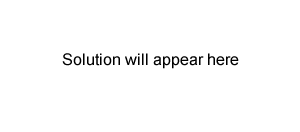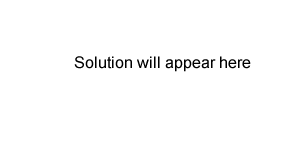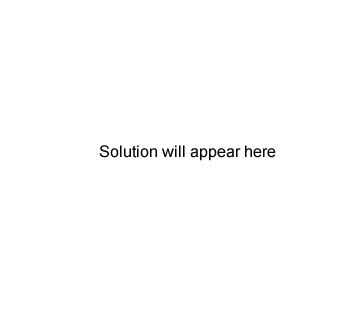Back titration questions-2008 0.415 g of a pure acid, H2X(s), is added to exactly 100 mL of 0.105 M NaOH(aq). A reaction occurs according to the equation H2X(s) + 2NaOH(aq) → Na2X(aq) + 2H2O(l) The NaOH is in excess. This excess NaOH requires 25.21 mL of 0.197 M HCl(aq) for neutralisation. Calculate i. the amount, in mol, of NaOH that is added to the acid H2X initially. Solutionii. the amount, in mol, of NaOH that reacts with the acid H2X. Answer is C Answer is D Radio wave frequencies are used in nuclear magnetic resonance spectroscopy in which the nuclei of C or H atoms are promoted to higher energy levels (option D). Option A indicated mass spectroscopy, option B indicated IR spectroscopy, and option C indicated atomic absorption or UV-Visible spectroscopy Solutioniii. the molar mass, in g mol–1, of the acid H2X. Solution